2019-09-28 12:43:55 duxinshuxiaobian 阅读数 1099
• ###### 机器学习&深度学习系统实战！

购买课程后，可扫码进入学习群，获取唐宇迪老师答疑 数学原理推导与案例实战紧密结合，由机器学习经典算法过度到深度学习的世界，结合深度学习两大主流框架Caffe与Tensorflow，选择经典项目实战人脸检测与验证码识别。原理推导，形象解读，案例实战缺一不可！具体课程内容涉及回归算法原理推导、决策树与随机森林、实战样本不均衡数据解决方案、支持向量机、Xgboost集成算法、神经网络基础、神经网络整体架构、卷积神经网络、深度学习框架--Tensorflow实战、案例实战--验证码识别、案例实战--人脸检测。 专属会员卡优惠链接：http://edu.csdn.net/lecturer/1079

40550 人正在学习 去看看 唐宇迪Scratch的深度学习实现

# Inputs and outputs of the neural net:import numpy as
npX=np.array([[1.0, 0.0, 1.0, 0.0],[1.0, 0.0, 1.0, 1.0],[0.0, 1.0, 0.0,
1.0]])yt=np.array([[1.0],[1.0],[0.0]])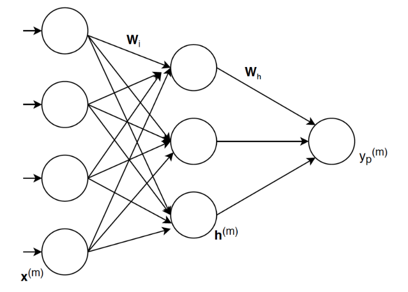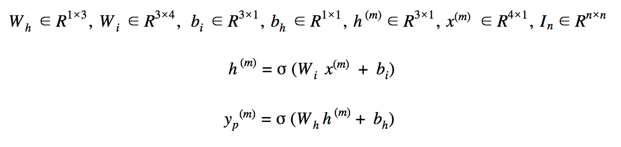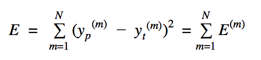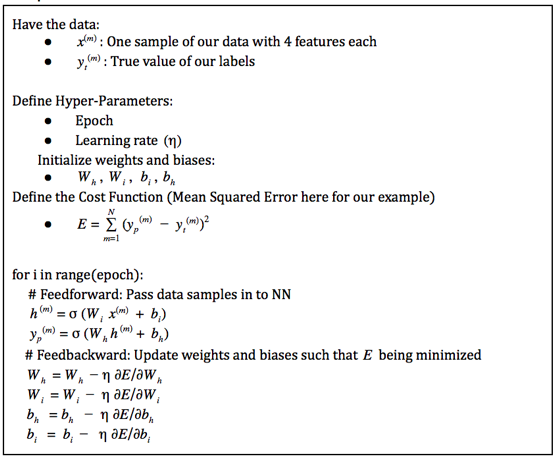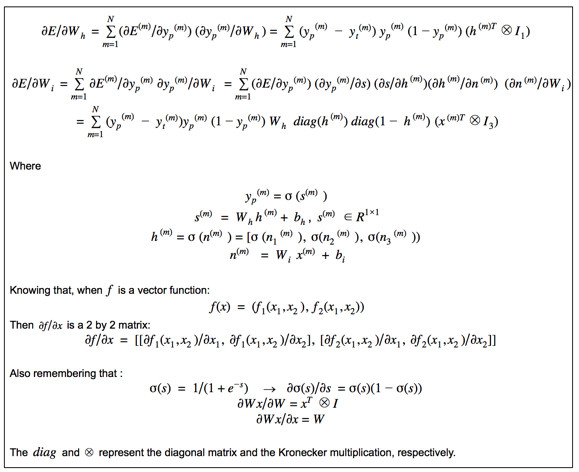1. 使用训练数据的全部个样本

2. 使用一个样本或一簇样本Keras、Tensorflow 1.x及2.0的深度学习实现

1. 高级（Keras和Tensorflow 2.0）：

2. 中级（Tensorflow 1.x和2.0）：

3. 初级（Tensorflow 1.x）：

# High-Level implementation of the neural net
in Tensorflow:model.compile(loss=mse,optimizer=optimizer)for _ in
range(2000):for step, (x, y) in enumerate(zip(X_data,
y_data)):model.train_on_batch(np.array([x]), np.array([y]))

E = tf.reduce_sum(tf.pow(ypred - Y, 2))optimizer =
optimizer.apply_gradients(grads)

# Medium-Level implementation of the neural net in Tensorflow#

In for_loop

with tf.GradientTape() as tape:   x =
tf.convert_to_tensor(np.array([x]), dtype=tf.float64)   y =
tf.convert_to_tensor(np.array([y]), dtype=tf.float64)   ypred =
rainable_weights))

# Low-Level implementation of the neural net in Tensorflow:E =
tf.reduce_sum(tf.pow(ypred - Y, 2))dE_dW_h = tf.gradients(E, [W_h])
dE_db_o = tf.gradients(E, [b_o])# In for_loop:evaluated_dE_dW_h =
sess.run(dE_dW_h,                 feed_dict={W_h: W_h_i, b_h: b_h_i,
W_o: W_o_i, b_o: b_o_i, X: X_data.T, Y: y_data.T})            W_h_i =
W_h_i - 0.1 * evaluated_dE_dW_h            evaluated_dE_db_h =
sess.run(dE_db_h,                             feed_dict={W_h: W_h_i,
b_h: b_h_i, W_o: W_o_i, b_o: b_o_i, X: X_data.T, Y: y_data.T})
b_h_i = b_h_i - 0.1 * evaluated_dE_db_h            evaluated_dE_dW_o
= sess.run(dE_dW_o,                             feed_dict={W_h: W_h_i,
b_h: b_h_i, W_o: W_o_i, b_o: b_o_i, X: X_data.T, Y: y_data.T})
W_o_i = W_o_i - 0.1 * evaluated_dE_dW_o            evaluated_dE_db_o
= sess.run(dE_db_o,                            feed_dict={W_h: W_h_i, b_h: b_h_i,
W_o: W_o_i, b_o: b_o_i, X: X_data.T, Y: y_data.T})
b_o_i = b_o_i - 0.1 * evaluated_dE_db_o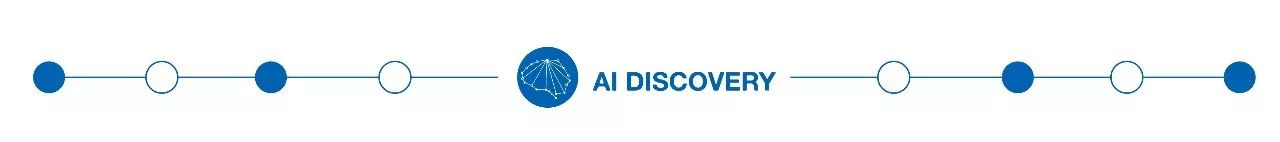（添加小编微信：dxsxbb，加入读者圈，一起讨论最新鲜的人工智能科技哦～）

2017-09-27 00:00:00 FnqTyr45 阅读数 2137
• ###### 机器学习&深度学习系统实战！

购买课程后，可扫码进入学习群，获取唐宇迪老师答疑 数学原理推导与案例实战紧密结合，由机器学习经典算法过度到深度学习的世界，结合深度学习两大主流框架Caffe与Tensorflow，选择经典项目实战人脸检测与验证码识别。原理推导，形象解读，案例实战缺一不可！具体课程内容涉及回归算法原理推导、决策树与随机森林、实战样本不均衡数据解决方案、支持向量机、Xgboost集成算法、神经网络基础、神经网络整体架构、卷积神经网络、深度学习框架--Tensorflow实战、案例实战--验证码识别、案例实战--人脸检测。 专属会员卡优惠链接：http://edu.csdn.net/lecturer/1079

40550 人正在学习 去看看 唐宇迪“王小二卖猪”解读深度学习之导数## 绕了半天，终于该求偏导出场了。目前的情况是：End.

--------------------2019-08-19 15:35:35 duxinshuxiaobian 阅读数 113
• ###### 机器学习&深度学习系统实战！

购买课程后，可扫码进入学习群，获取唐宇迪老师答疑 数学原理推导与案例实战紧密结合，由机器学习经典算法过度到深度学习的世界，结合深度学习两大主流框架Caffe与Tensorflow，选择经典项目实战人脸检测与验证码识别。原理推导，形象解读，案例实战缺一不可！具体课程内容涉及回归算法原理推导、决策树与随机森林、实战样本不均衡数据解决方案、支持向量机、Xgboost集成算法、神经网络基础、神经网络整体架构、卷积神经网络、深度学习框架--Tensorflow实战、案例实战--验证码识别、案例实战--人脸检测。 专属会员卡优惠链接：http://edu.csdn.net/lecturer/1079

40550 人正在学习 去看看 唐宇迪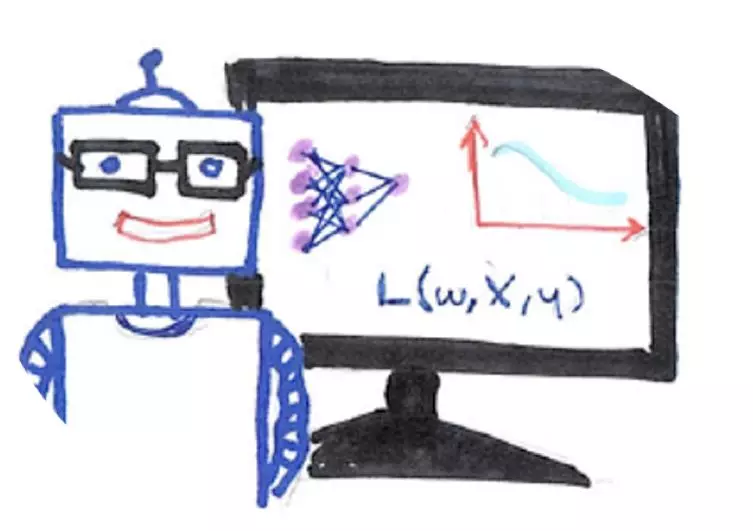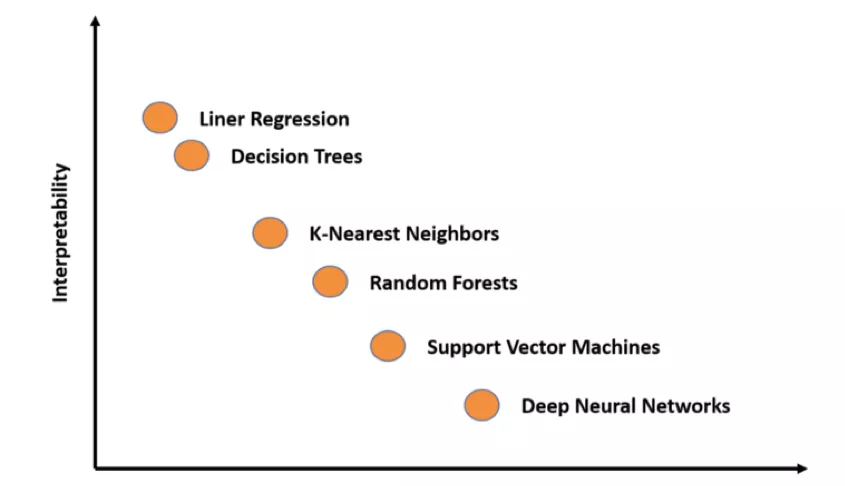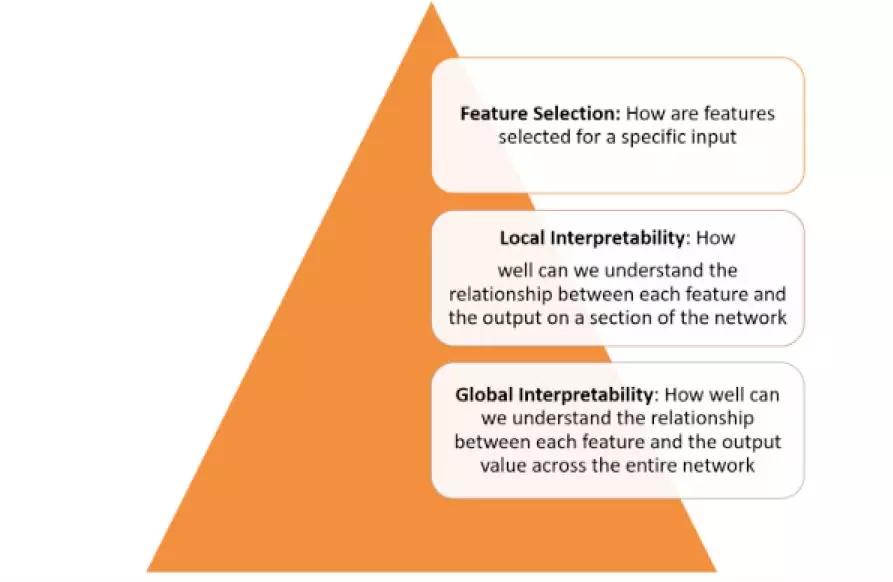-明白隐藏层的作用：深度学习模型中的许多原理都是在隐藏层中实现的。宏观了解各个隐藏层功能对解释深度学习模型而言至关重要。

-明白节点激活方式：可解释性的关键不是理解单个神经元在网络中的功能，而在于明白相同空间位置中互相联系的各组神经元之间的作用。在拆分神经网络之时，对相互关联的神经元分组将有助于理解该网络的功能。

-明白概念形成过程：了解深度神经中枢网络形成自身概念进而得出最终结果的过程是建立可解释性的另一关键。-可行性：使用者几乎无需具备深度学习知识

-定制性：适配于任何概念（如性别等）且不仅限于训练时使用的概念

-内嵌可读性：无需深度学习模型再次训练或优化。

-全球定量：可使用单个定量度量来解释整类或整组示例，而非解释单一数据输入。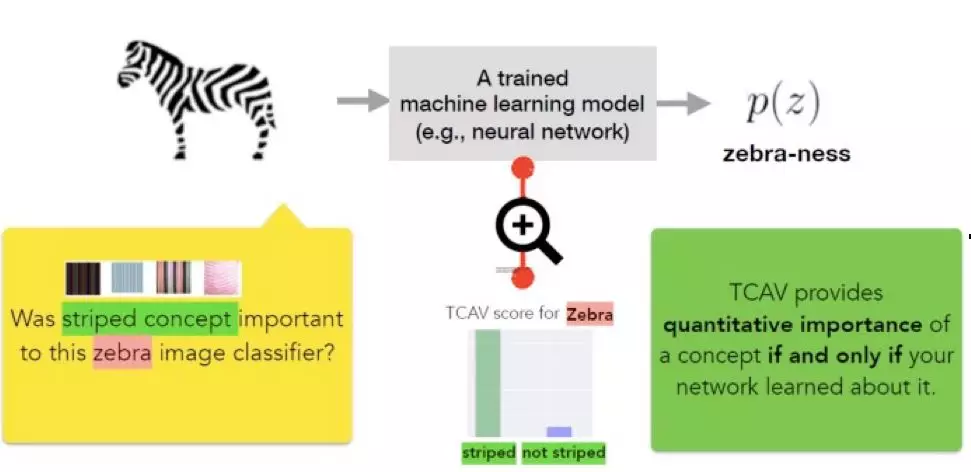1）定义模型的相关概念。

2）理解这些概念预测结果的敏感性。

3）推断各概念对各模型预测类相对重要性，做出全局定量解释。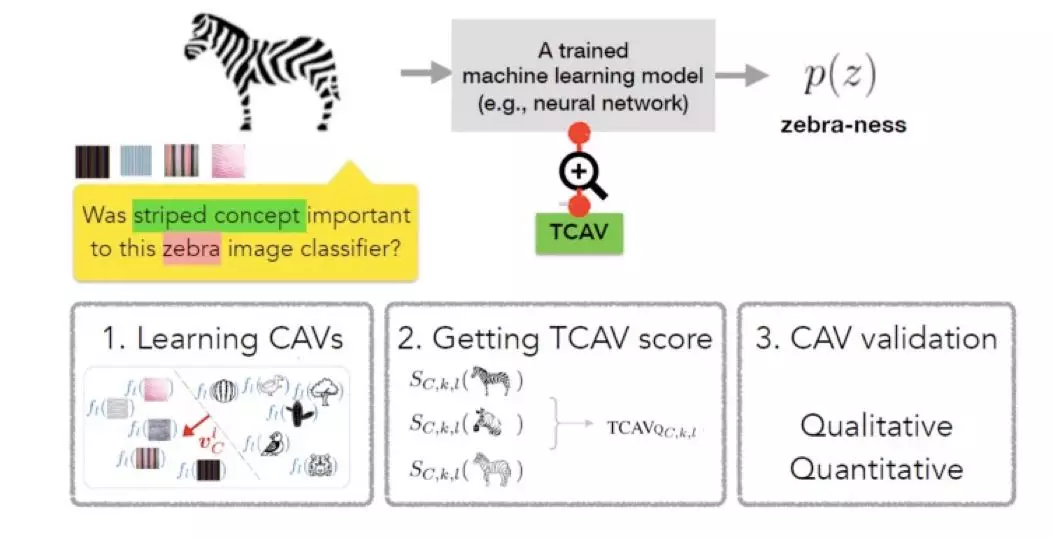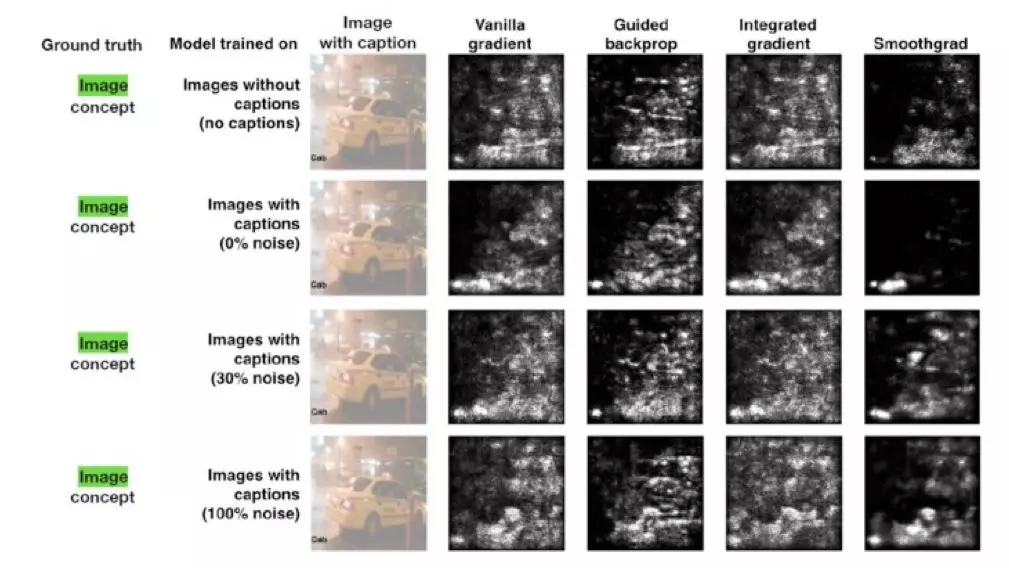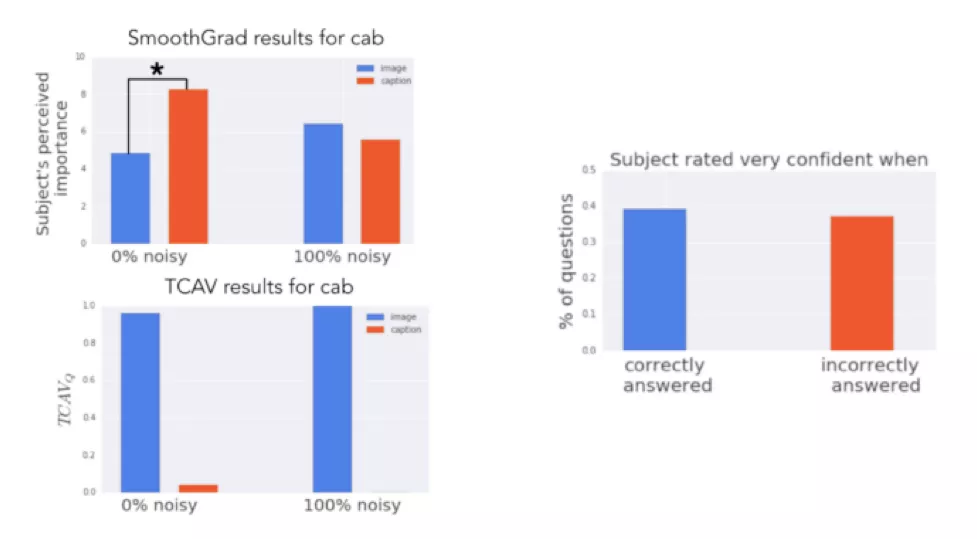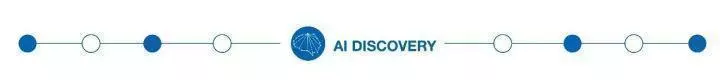（添加小编微信：dxsxbb，加入读者圈，一起讨论最新鲜的人工智能科技哦～）

2018-01-14 14:08:39 xbinworld 阅读数 24498
• ###### 机器学习&深度学习系统实战！

购买课程后，可扫码进入学习群，获取唐宇迪老师答疑 数学原理推导与案例实战紧密结合，由机器学习经典算法过度到深度学习的世界，结合深度学习两大主流框架Caffe与Tensorflow，选择经典项目实战人脸检测与验证码识别。原理推导，形象解读，案例实战缺一不可！具体课程内容涉及回归算法原理推导、决策树与随机森林、实战样本不均衡数据解决方案、支持向量机、Xgboost集成算法、神经网络基础、神经网络整体架构、卷积神经网络、深度学习框架--Tensorflow实战、案例实战--验证码识别、案例实战--人脸检测。 专属会员卡优惠链接：http://edu.csdn.net/lecturer/1079

40550 人正在学习 去看看 唐宇迪

## 1.线性代数

1.1 标量，向量，矩阵和张量

• 标量（scalar）：一个标量就是一个单独的数。用斜体表示标量，如 sR$s\in R$.
• 向量（vector）：一个向量是一列数，我们用粗体的小写名称表示向量。比如
x，将向量x 写成方括号包含的纵柱：

x=x1x2xn

• 矩阵（matrix）：矩阵是二维数组，我们通常赋予矩阵粗体大写变量名称，比如
A​ 。如果一个矩阵高度是m​，宽度是n​，那么说ARm×n$A \in R^{m×n}$ 。一个矩阵可以表示如下：

A=[x11x21x12x22]

• 张量（tensor）：某些情况下，我们会讨论不止维坐标的数组。如果一组数组中的元素分布在若干维坐标的规则网络中，就将其称为张量。用A​ 表示，如张量中坐标为(i,j,k)​的元素记作Ai,j,k$A_{i,j,k}$

• 转置（transpose）：矩阵的转置是以对角线为轴的镜像，这条从左上角到右下角的对角线称为主对角线（main diagonal）。将矩阵A的转置表示为AT$A^T$。定义如下： (A)i,j=Aj,i$({\bf A^\top})_{i,j}=\bf A_{j,i}$

A=x11x21x31x12x22x32A=[x11x21x21x22x31x32]

1.2 矩阵和向量相乘

• 矩阵乘法：矩阵运算中最重要的操作之一。两个矩阵A和B 的矩阵乘积(matrix product)是第三个矩阵C 。矩阵乘法中A 的列必须和 B的行数相同。即如果矩阵 A的形状是 m×n，矩阵B的形状是 n×p，那么矩阵 C的形状就是 m×p。即

C=A×B
具体的地，其中的乘法操作定义为

Ci,j=kAi,kBk,j
矩阵乘积服从分配律，结合律，但是矩阵乘法没有交换律，

A(B+C)=AB+ACA(BC)=(AB)CABBA

• 点积（dot product）两个相同维数的向量x和y的点积可看作是矩阵乘积 xTy$x^Ty$

• 矩阵乘积的转置 (向量也是一种特殊的矩阵)

(AB)=BAxy=(xy)=yx

1.3 单位矩阵和逆矩阵

A1A=AA1=InInA=A

1.4 范数

||x||p=(i|xi|p)1ppR,p1

f(x)=0x=0f(x+y)f(x)+f(y)αR,f(αx)=|α|f(x)

max范数（max norm）：这个范数表示向量中具有最大幅度得元素的绝对值，用L$L_\infty$范数表示，形式为：

||x||=max|xi|

xy=||x||2||y||2cosθ

||A||F=(ij|aij|2)1/2

1.5 特殊类型的矩阵和向量

• 对角矩阵 (diagonal matrix) 只在主对角线上含有非零元素，其它位置都是零。矩阵D是对角矩阵，当且仅当 ∀i≠j,Di,j=0，用 diag(v)表示一个对角元素由向量v中元素给定的对角矩。对称(symmetric) 矩阵是任意转置和自己相等的矩阵： A=A$\bf A = A^ \top$.

• 单位向量（unit vector） 是具有单位范数（unit norm） 的向量：||x||2=1$||{\bf x}||_2 = 1$

• 正交矩阵（orthonormal matrix） 是指行向量是标准正交的，列向量是标准正交的方阵：
如果xy=0$x^⊤y=0$，那么向量 x和向量 y互相正交（orthogonal）。如果两个向量都有非零范数，那么表示这两个向量之间的夹角是90度。在Rn$R^n$ 中，至多有n个范数非零向量互相正交。如果这些向量不仅互相正交，并且范数都为1那么我们称它们是标准正交（orthonormal） 。
AA=AA=IA1=A

所以正交矩阵受到关注是因为求逆计算代价小。

1.6 特征分解

Av=λv

V={v1,v2,...,vn}

λ=[λ1,λ2,...,λn]

A=Vdiag(λ)V1

VVT=VTV=I

A=VΛVT=[v1,v2,,vn]λ1λnvT1vT2vTn=i=1nλivivTi1.7 矩阵的迹

Tr(A)=iAi,i

||A||F=Tr(AA)

Tr(A)=Tr(A)

Tr(ABC)=Tr(BCA)=Tr(CAB)

xTAx=xixjaij

## 2. 矩阵求导， 凸函数

2019-05-18 23:08:19 LXYTSOS 阅读数 214
• ###### 机器学习&深度学习系统实战！

购买课程后，可扫码进入学习群，获取唐宇迪老师答疑 数学原理推导与案例实战紧密结合，由机器学习经典算法过度到深度学习的世界，结合深度学习两大主流框架Caffe与Tensorflow，选择经典项目实战人脸检测与验证码识别。原理推导，形象解读，案例实战缺一不可！具体课程内容涉及回归算法原理推导、决策树与随机森林、实战样本不均衡数据解决方案、支持向量机、Xgboost集成算法、神经网络基础、神经网络整体架构、卷积神经网络、深度学习框架--Tensorflow实战、案例实战--验证码识别、案例实战--人脸检测。 专属会员卡优惠链接：http://edu.csdn.net/lecturer/1079

40550 人正在学习 去看看 唐宇迪• 理解Pytorch 的 Tensor库，以及神经网络。
• 训练一个简单的图像分类网络。

# 什么是Pytorch

Pytorch是一个基于Python的科学计算包，用于以下两个目的：

1. 代替NumPy，使用GPU的加速能力。
2. 用于提供最大灵活性和速度的深度学习研究平台。

## 张量（Tensors）

x = torch.empty(5, 3)
print(x)


tensor([[0.0000e+00, 0.0000e+00, 0.0000e+00],
[0.0000e+00, 0.0000e+00, 0.0000e+00],
[0.0000e+00, 0.0000e+00, 0.0000e+00],
[0.0000e+00, 7.6231e-43, 0.0000e+00],
[0.0000e+00, 2.9966e+32, 0.0000e+00]])


x = torch.rand(5, 3)
print(x)


tensor([[0.4140, 0.7152, 0.0888],
[0.6878, 0.9607, 0.2579],
[0.0088, 0.5816, 0.1782],
[0.3815, 0.6752, 0.9996],
[0.4057, 0.3032, 0.8977]])


x = torch.zeros(5, 3, dtype=torch.long)
print(x)


tensor([[0, 0, 0],
[0, 0, 0],
[0, 0, 0],
[0, 0, 0],
[0, 0, 0]])


x = torch.tensor([5.5, 3])
print(x)


tensor([5.5000, 3.0000])


x = x.new_ones(5, 3, dtype=torch.double)      # new_* 方法接收tensor大小参数
print(x)

x = torch.randn_like(x, dtype=torch.float)    # 重载数据类型!
print(x)                                      # 结果有相同的大小


tensor([[1., 1., 1.],
[1., 1., 1.],
[1., 1., 1.],
[1., 1., 1.],
[1., 1., 1.]], dtype=torch.float64)
tensor([[ 0.6743, -0.3788,  0.7561],
[-0.7612, -1.6943, -1.2451],
[ 0.6169, -1.1207, -1.1664],
[ 0.4309,  1.3055, -0.0481],
[ 0.7891, -1.7696,  1.4875]])


print(x.size())


torch.Size([5, 3])


## 操作（Operations）

y = torch.rand(5, 3)
print(x + y)


tensor([[1.2953, 0.3779, 0.9404],
[0.8671, 0.5968, 0.7860],
[1.3698, 1.2983, 1.1537],
[0.3706, 0.6296, 0.7085],
[0.8819, 0.8260, 0.9962]])


print(torch.add(x, y))


tensor([[1.2953, 0.3779, 0.9404],
[0.8671, 0.5968, 0.7860],
[1.3698, 1.2983, 1.1537],
[0.3706, 0.6296, 0.7085],
[0.8819, 0.8260, 0.9962]])


result = torch.empty(5, 3)
print(result)


tensor([[1.2953, 0.3779, 0.9404],
[0.8671, 0.5968, 0.7860],
[1.3698, 1.2983, 1.1537],
[0.3706, 0.6296, 0.7085],
[0.8819, 0.8260, 0.9962]])


# 把x加在y上
print(y)


tensor([[1.2953, 0.3779, 0.9404],
[0.8671, 0.5968, 0.7860],
[1.3698, 1.2983, 1.1537],
[0.3706, 0.6296, 0.7085],
[0.8819, 0.8260, 0.9962]])


print(x[:, 1])


tensor([0.1801, 0.1028, 0.7038, 0.3089, 0.1738])


x = torch.randn(4, 4)
y = x.view(16)
z = x.view(-1, 8)  # -1表示这个维度的数值通过其他维度推算而出
print(x.size(), y.size(), z.size())


torch.Size([4, 4]) torch.Size() torch.Size([2, 8])


x = torch.randn(1)
print(x)
print(x.item())


tensor([0.3665])
0.36647817492485046


# 对接NumPy

Torch Tensor和NumPy会共享数据底层的内存存储位置，所以修改其中一个也会改变另外一个的值。

Torch Tensor转成NumPy数组：

a = torch.ones(5)
print(a)


tensor([1., 1., 1., 1., 1.])

b = a.numpy()
print(b)


[1. 1. 1. 1. 1.]


a.add_(1)
print(a)
print(b)


tensor([2., 2., 2., 2., 2.])
[2. 2. 2. 2. 2.]


NumPy数组转Torch Tensor

import numpy as np
a = np.ones(5)
b = torch.from_numpy(a)
print(a)
print(b)


[2. 2. 2. 2. 2.]
tensor([2., 2., 2., 2., 2.], dtype=torch.float64)


CPU上的Tensor，除类型为CharTensor之外，都能和NumPy互转。

## CUDA Tensors

# 如果支持CUDA设备
# 使用 torch.device 将tensor移入或移出GPU
if torch.cuda.is_available():
device = torch.device("cuda")          # CUDA设备对象
y = torch.ones_like(x, device=device)  # 直接在GPU上创建
x = x.to(device)                       # 或者使用 .to("cuda")将tensor放到GPU上
z = x + y
print(z)
print(z.to("cpu", torch.double))       # .to 也能同时修改数据类型!


tensor([-0.7816], device='cuda:0')
tensor([-0.7816], dtype=torch.float64)